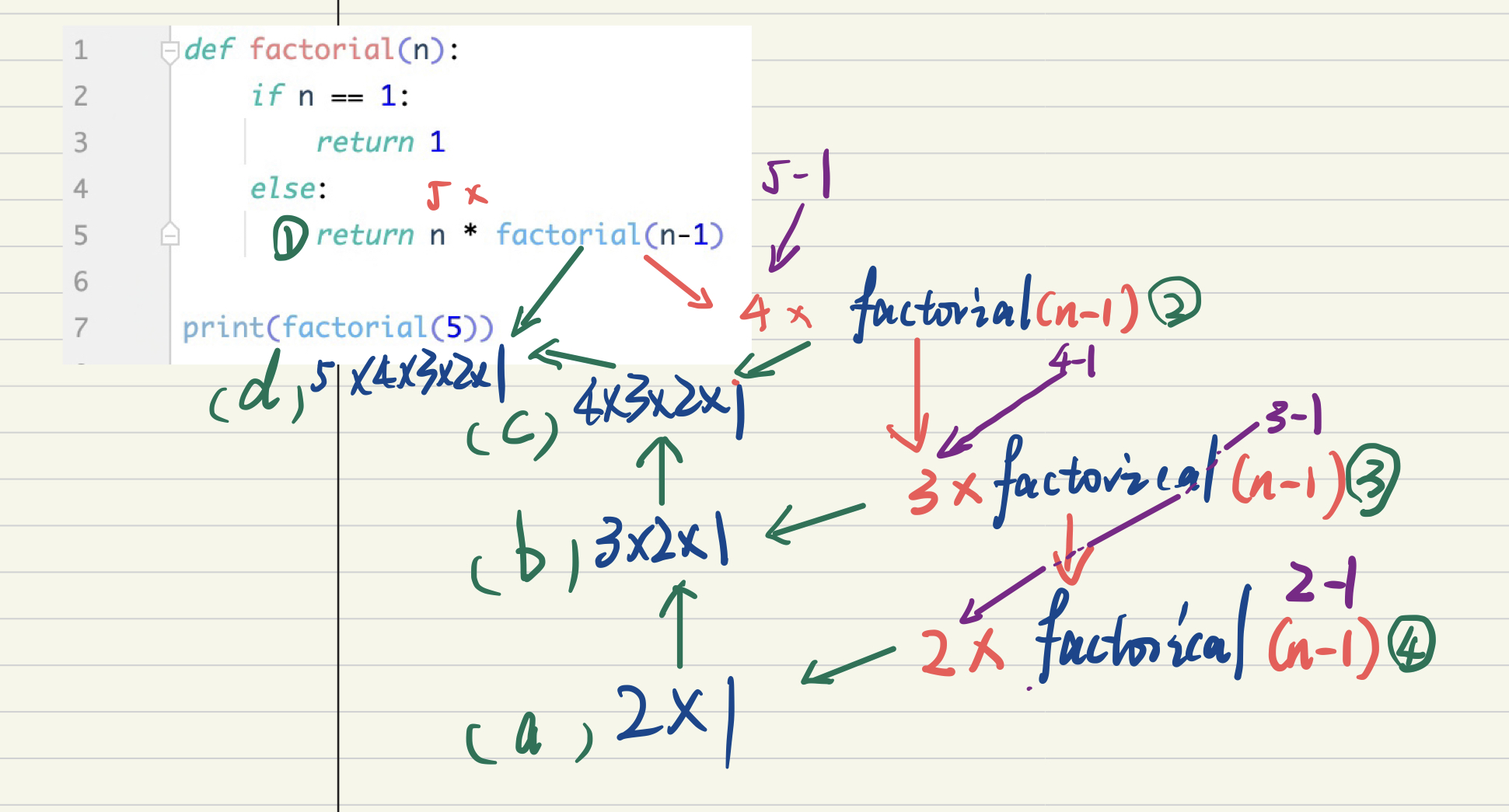> 文档中心 > Python递归思想与代码实现

# Python递归思想与代码实现

## 1， 递归思想

• 函数内部自己调用自己
• 函数必须有出口

``def recursion(n):    print(n, "")    if n > 0: recursion(n - 1)    # 那么这就是一个出口，如果n <= 0了就会不再递归    else: print(n, '')recursion(5) # 外部调用``

``5 4 3 2 1 0 0   # 这个是出口，在这个时候n = 0Process finished with exit code 0``

1. 递推：像上边递归实现所拆解，递归每一次都是基于上一次进行下一次的执行，这叫递推。
2. 回溯：则是在遇到终止条件，则从最后往回返一级一级的把值返回来，这叫回溯。

## 2，递归求阶乘，代码实现与思想解析

``def factorial(n):    # 出口    if n == 1: return 1    else: return n * factorial(n-1)print(factorial(5))``n * factorial(n-1)是等价表达式。红色箭头是递推的过程，从1-2-3-4；绿色箭头是回溯的过程，从a-b-c-d；紫色箭头是参数的变化。我把每一个式子都分解了一下，我们可以发现最后真正输出的是最外层的return，回溯顺序是从最底层往上返回。

``def factorial(n):    # 出口    if n == 1: return 1    # 递归内层    else: factor = n * factorial(n-1) print(factor) return factorprint(factorial(5))``

``2624120120Process finished with exit code 0``

``# 求5的阶乘num = 1for i in range(1,6):    num *= iprint(num)``

## 3，栈溢出

``import sys# 得到最大的深度值print(sys.getrecursionlimit())# 设置最大深度为2000sys.setrecursionlimit(2000)``

## 4，运行速度

``import sysimport timeprint(sys.getrecursionlimit())sys.setrecursionlimit(2000)start = time.time()def factorial(n):    # 出口    if n == 1: return 1    # 递归内层    else: return n * factorial(n-1)print(factorial(1000))print(time.time() - start)``

1. 递归由于是函数调用自身，而函数调用是有时间和空间的消耗的--效率
2. 递归中很多计算都是重复的，由于其本质是把一个问题分解成两个或者多个小问题，多个小问题存在相互重叠的部分，则存在重复计算--效率
3. 调用栈可能会溢出，其实每一次函数调用会在内存栈中分配空间，而每个进程的栈的容量是有限的，当调用的层次太多时，就会超出栈的容量，从而导致栈溢出，强制设置最大深度会导致内存占用大--性能

1. 简洁
2. 在特殊情况下比for循环更加简洁，逻辑清晰。